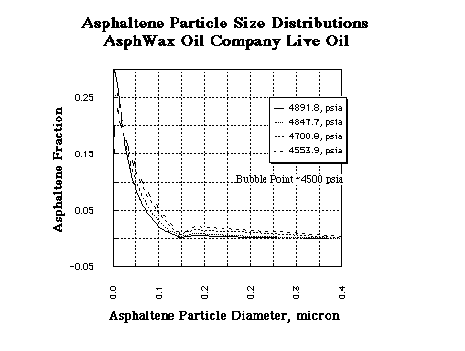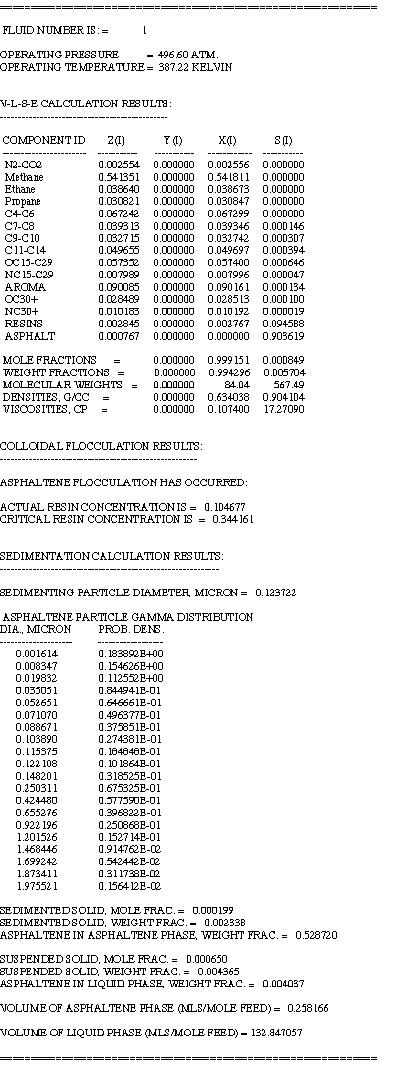TCModelR by AsphWax

Asphaltene Phase Behavior Simulator

The Thermodynamic-Colloidal Model of Asphaltenes (TCModelR) computer program is capable of simulating the asphaltene phase behavior of reservoir fluids. The TCModelR can calculate the complete asphaltene deposition envelope (ADE) of a reservoir fluid. The TCModelR is the only model available on the market capable of calculating the particle size distribution of flocculated asphaltenes as a function of the thermodynamics of the system.The figure shown below shows a plot of four asphaltene particle size distributions calculated by the TCModelR at reservoir temperature and four pressures (4891.8, 4847.7, 4700.8, and 4553.9 psia) inside the upper ADE (i.e., no gas present) of another reservoir fluid. The plot shows that, as the pressure drops and approaches the bubble point (at about 4500 psia), the asphaltene psd moves to the right, i.e., the mean of the asphaltene psd increases as the pressure drops.An example output of the TCModelR for a calculation inside the deposition envelope and above the bubble point line is shown below.An example output of the TCModelR for a complete P-T Diagram and asphaltene deposition envelope calculation is shown below.